Dedicated to those who never had the opportunity to receive a good education
Dedicated to those who never had the opportunity to receive a good education
0%

# Maxwell equations in matter

The Maxwell equations are a set of four partial differential equations that describe the spatial and temporal behavior of electric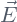and magnetic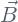fields. The sources of time-independent electric and magnetic fields are the time-independent electric charge density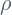and the steady current density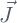respectively. The study of electric and magnetic phenomena that do not vary in time are called electrostatic and magnetostatic respectively.

When the spatial distribution of charges and currents are time-dependent new phenomena take place. In a time-varying situation, the electric and magnetic fields depend on time and their temporal derivatives become sources of the electromagnetic field besidesand.

The electric and magnetic fields are vector quantities. A mathematical theorem due to Helmholtz asserts that to completely determine a vector field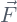, it is enough to specify its divergence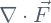, and its curl (or rotational)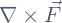, whenever these quantities decrease to zero sufficiently rapid at infinity. Maxwell equations precisely specify the divergence and rotational of the electric and magnetic fields in terms of their sources.

## General form of Maxwell equations

Let’s recall here the Maxwell equations:

(1)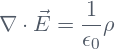(2)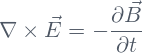(3)(4)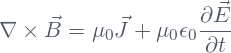The charge densitycan be written as the sum of two contributions, namely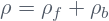, where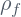is the density of free charges, while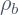is the density of bound charges. The bound charge density is related to the polarization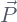of the medium through the equation

(5)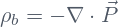Let’s see how Eq.(5) is obtained

## Relation between bound charge density and polarization

The relation given in Eq.(5) is not an apriori definition. It is derived by considering  the electrostatic potential due to a spatial distribution of pure dipoles. We recall here the electrostatic potential due to a single electric dipole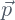, namely

(6)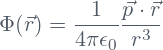The polarizationis the density of dipoles defined as the vector sum of pure dipoles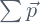contained in an infinitesimal volume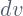divided by that infinitesimal volume. Therefore,  the expression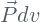represents the net dipole resulting of the vector sum of all of the dipoles contained in the infinitesimal element of volume. The electrostatic potential due to the polarizationis therefore

(7)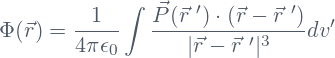Now we use the identity

(8)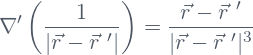inside the integral which becomes

(9)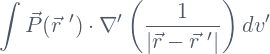In the preceding equations,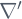means derivation with respect to the primed coordinates. The Leibnitz’ rule allows us to rewrite the integrand in Eq.(9) in the form

(10)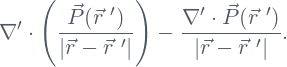This implies that the electrostatic potential can be written as the sum of two terms, namely

(11)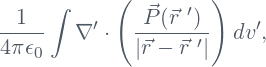and

(12)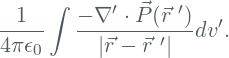The contribution to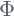coming from Eq.(12) looks like the potential due to a charge distribution whose density is precisely given by Eq.(5).

The volume integral in Eq.(11) can be transformed to a surface integral with the help of the divergence theorem, namely

(13)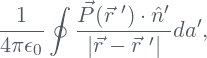where the integral extends over the surface of the body. The contribution tocoming from Eq.(13) looks like the potential due to a surface charge distribution whose density is

(14)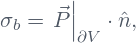where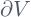denotes the surface of the volume occupied by the body and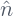is the unit vector normal to the surface.

The surface charge density cannot be used with the differential form of the Gauss’ law Eq.(1) which is valid inside the body only. Nevertheless, the no inclusion of the surface charge density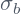induced by the polarization, in the definition of the bound charge density, does not represent a problem. Indeed, the integral form of Gauss’ law is valid everywhere and the surface charge density does not contribute either (this is so because a volume integral has the same value independently of whether the boundary is included or not).

## Gauss’ law inside matter

The first of Maxwell equations, Eq.(1) is the differential form of Gauss law. In terms of the free and bound charge densities it can be rewritten as follows: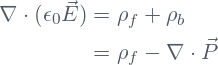Or, equivalently

(15)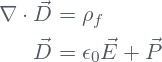The partial differential equation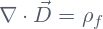is identical to the Gauss law given in Eq.(1). It just has been written in a form that makes explicit the fact that the medium responds to the electric field by becoming polarized. How exactly the polarizationdepends on the electric fieldis a problem that has to be analyzed separately.

## Ampere’s law inside matter

Ampere’s law with the modification made by Maxwell to include the effect on the magnetic fielddue to a time varying electric field, is given in Eq.(4). The current density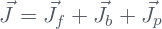has three contributions coming from free charges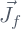, bound charges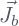, and polarization effects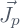. The bound current densityis related to the magnetization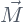of the medium through the equation

(16)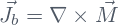We will not derive here the relation given in Eq.(16), but we remark that it is obtained in a similar way to the derivation of Eq.(5) in the case of magnetostatic. The polarization current densityhas its origin in the continuous displacement of bound charges in response to a time varying electric field. The continuous displacement of bound charges creates a time varying polarization. The equation relatingto the time varying polarization is

(17)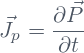The decomposition, together with Eq.(16) and Eq.(17), allows rewriting Ampere’s law Eq.(4) as follows:

(18)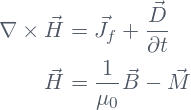Equations (2), (3), (15) and (18), are known as Maxwell equations in matter. Actually, they just make explicit that the material responds to electric and magnetic fields through polarization and magnetization. Note that Eq.(2) and Eq.(3), including no explicit relation with charges and currents, remain unaltered.

Share it!